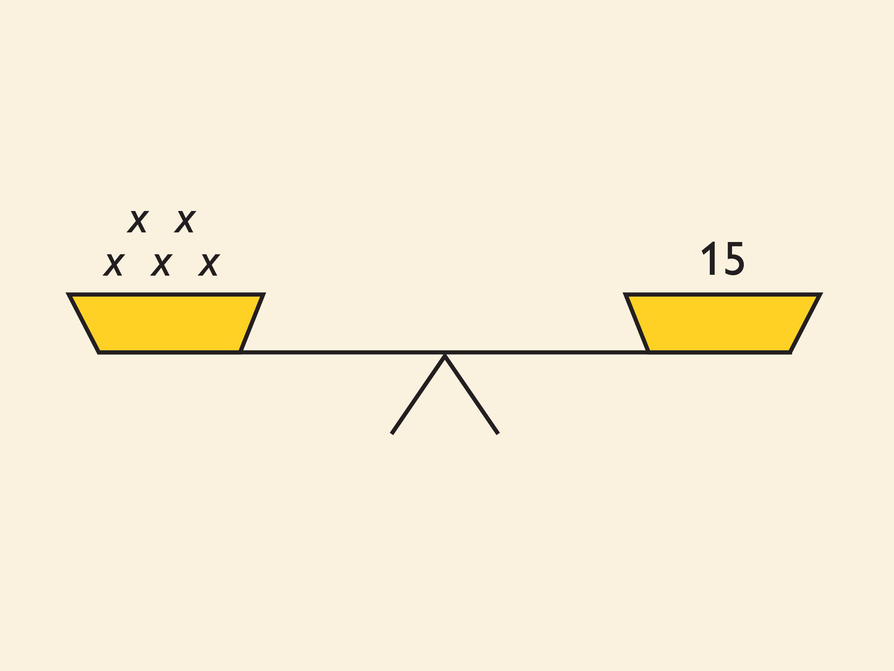# Solve for x

Look at the balance scale.

• Write an equation that represents the balance scale.
• Can you multiply or divide both sides of the equation by some number in order to find the value of x that makes the equation true?
• What is the solution?
• Substitute your solution for x in your equation to check your answer.